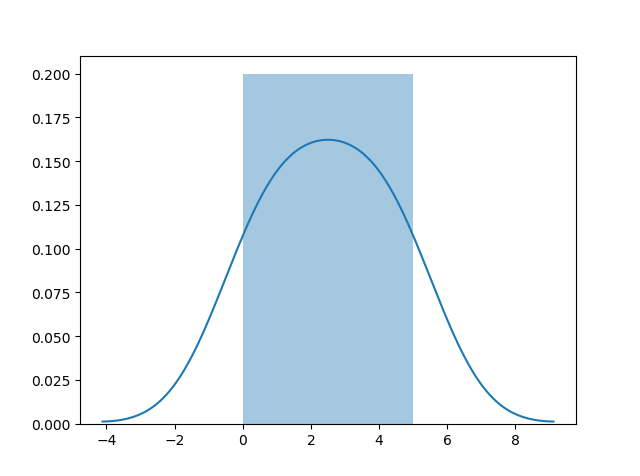# Seaborn

## Visualize Distributions With Seaborn

Seaborn is a library that uses Matplotlib underneath to plot graphs. It will be used to visualize random distributions.## Install Seaborn.

If you have Python and PIP already installed on a system, install it using this command:

If you use Jupyter, install Seaborn using this command:

## Distplots

Distplot stands for distribution plot, it takes as input an array and plots a curve corresponding to the distribution of points in the array.

## Import Matplotlib

Import the pyplot object of the Matplotlib module in your code using the following statement:
import matplotlib.pyplot as plt

You can learn about the Matplotlib module in our Matplotlib Tutorial.

## Import Seaborn

Import the Seaborn module in your code using the following statement:
import seaborn as sns

## Plotting a Distplot

### Example

import matplotlib.pyplot as plt
import seaborn as sns

sns.distplot([0, 1, 2, 3, 4, 5])

plt.show()
Try it Yourself »

## Plotting a Distplot Without the Histogram

### Example

import matplotlib.pyplot as plt
import seaborn as sns

sns.distplot([0, 1, 2, 3, 4, 5], hist=False)

plt.show()
Try it Yourself »

Note: We will be using: `sns.distplot(arr, hist=False)` to visualize random distributions in this tutorial.

W3Schools is optimized for learning and training. Examples might be simplified to improve reading and learning. Tutorials, references, and examples are constantly reviewed to avoid errors, but we cannot warrant full correctness of all content. While using W3Schools, you agree to have read and accepted our terms of use, cookie and privacy policy.